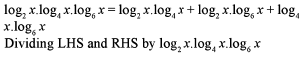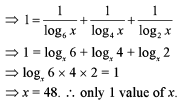Courses

# Test: Logarithm- 3

## 15 Questions MCQ Test Quantitative Aptitude (Quant) | Test: Logarithm- 3

Description
This mock test of Test: Logarithm- 3 for CAT helps you for every CAT entrance exam. This contains 15 Multiple Choice Questions for CAT Test: Logarithm- 3 (mcq) to study with solutions a complete question bank. The solved questions answers in this Test: Logarithm- 3 quiz give you a good mix of easy questions and tough questions. CAT students definitely take this Test: Logarithm- 3 exercise for a better result in the exam. You can find other Test: Logarithm- 3 extra questions, long questions & short questions for CAT on EduRev as well by searching above.
QUESTION: 1

### If 3X+I = 6log23 then x is

Solution: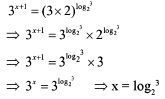QUESTION: 2

### log5P x logp x = 2. What is the value of x?

Solution: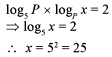QUESTION: 3

### If [N] = the greatest integer less than or equal to N, then [log10 6730.4] is equal to

Solution: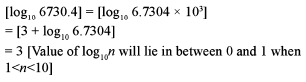QUESTION: 4

What is the number of zeros coming immediately after the decimal point in the value of (0.2)25 ? (log102 = 0.30103)

Solution: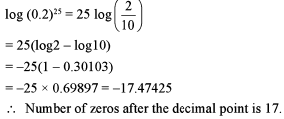QUESTION: 5

Find the value of log64(l/16).

Solution: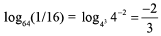QUESTION: 6

Arrange the following in an ascending order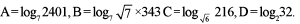Solution: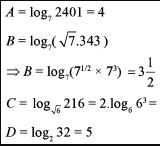A = 4, B = 7/2, C = 6, D = 5

QUESTION: 7

What is the value of x if log3x + log9x + log27x + log81x  = 25/4?

Solution: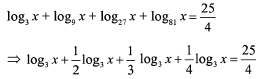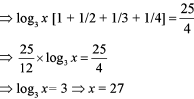QUESTION: 8

What is the value of x in the following expression: log2 ( 3 - x ) + log2 ( 1 - x ) = 3?

Solution:

Going through options.
Option (a) is not possible.
Checking option (c): Forx = -1, expression = log24 + log22 = log28 = 31og22 = 3

QUESTION: 9

What is the value of log3√3 27 ?

Solution: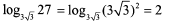QUESTION: 10

What is the value of P if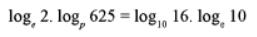?

Solution: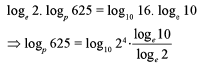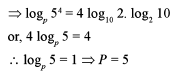QUESTION: 11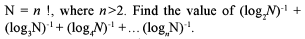Solution: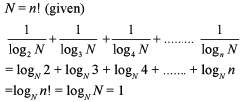QUESTION: 12

If x = loga (bc), y = log4 (ca) and z = logc (ab) when which of the following is equal to 1?

Solution: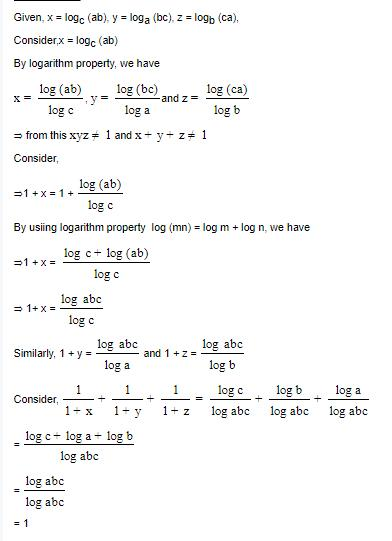QUESTION: 13

If logk x.log5 k = 1 k ≠ 1.k > 0, then x is equal to

Solution: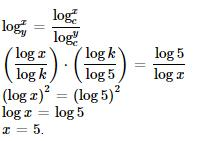QUESTION: 14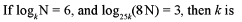Solution: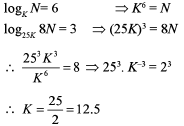QUESTION: 15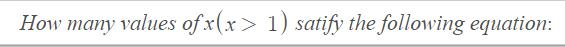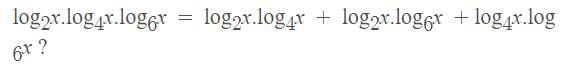Solution: